Diagrams with multiple metals

This vignette was compiled on 2021-04-08 with CHNOSZ version 1.4.1.

The plots in this vignette were made using the following resolution settings, which can be changed if desired (low resolutions are used to save time in CRAN checks):

res1 <- 150
# res1 <- 256
res2 <- 200
# res2 <- 400

Basic diagrams in CHNOSZ are made for reactions that are balanced on an element (see Equilibrium in CHNOSZ) and therefore represent minerals or aqueous species that all have one element, often a metal, in common. The package documentation has many examples of diagrams for a single metal appearing in different minerals or complexed with different ligands, but a common request is to make diagrams for multiple metals. This vignette describes some methods for constructing diagrams for multi-metal minerals and other multi-element systems. The methods are mashing, mixing, mosaic stacking, and secondary balancing.

Mashing

Mashing or simple overlay refers to independent calculations for two different systems that are displayed on the same diagram.

This example starts with a logfO2–pH base diagram for the C-O-H system then overlays a diagram for S-O-H. The second call to affinity() uses the argument recall feature, where the arguments after the first are taken from the previous command. This allows calculations to be run at the same conditions for a different system. This feature is also used in other examples in this vignette.

par(mfrow = c(1, 2))
basis("CHNOS+")
species(c("CH4", "CO2", "HCO3-", "CO3-2"))
aC <- affinity(pH = c(0, 14), O2 = c(-75, -60))
dC <- diagram(aC, dx = c(0, 1, 0, 0), dy = c(0, 1, 0, 0))
species(c("H2S", "HS-", "HSO4-", "SO4-2"))
aS <- affinity(aC)  # argument recall
dS <- diagram(aS, add = TRUE, col = 4, col.names = 4, dx = c(0, -0.5, 0, 0))

The second diagram is just like the first, except the function mash() is used to label the fields with names of species from both systems, and a legend is added to indicate the temperature and pressure.

aCS <- mash(dC, dS)
srt <- c(0, 0, 90, 0, 0, 0, 90, 0, 0, 0)
cex.names <- c(1, 1, 0.8, 1, 1, 1, 1, 1, 1, 1)
dy <- c(0, 0, 0, -0.2, 0, 0, 0, 0, 0, 0)
diagram(aCS, srt = srt, cex.names = cex.names, dy = dy)
legend("topright", legend = lTP(25, 1), bty = "n")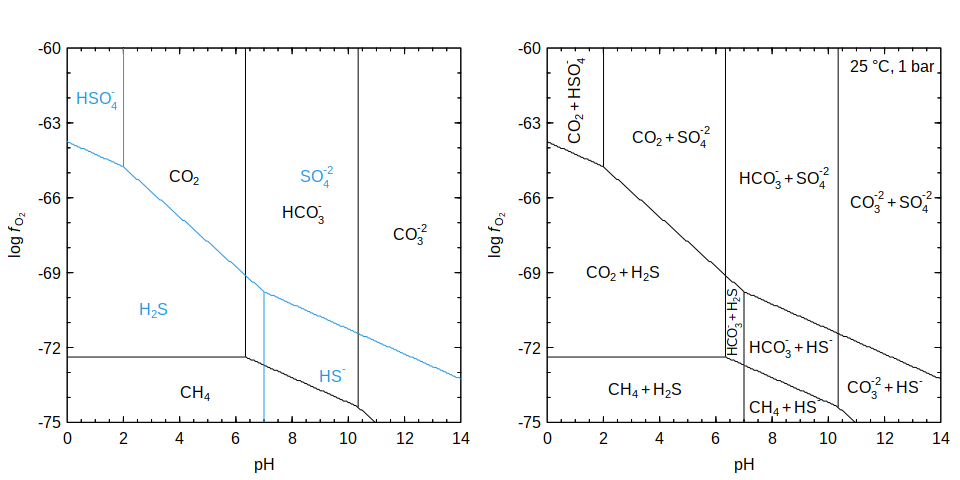Note that these are predominance diagrams, so they show only the species with highest activity; there is in fact a distribution of activities of aqueous species that is not visible here.

Tip: the names of the fields in the second diagram come from aCS\$species\$name, which are expressions made by combining aC\$names and aS\$names. If you prefer plain text names without formatting, add format.names = FALSE to all of the diagram() calls.

Mixing 1

As shown above, mashing two diagrams is essentially a simple combination of the two systems. Although it is easy to make such a diagram, there is no interaction between the systems. If there is a possibility of forming bimetallic species, then additional considerations are needed to account for the stoichiometry of the mixture. The stoichiometry can be given as a fixed composition of both metals; then, all combinations of (mono- and/or bimetallic) species that satisfy this compositional constraint are used as the candidate “species” in the system. This is the same type of calculation as that described for binary Pourbaix diagrams in the Materials Project.

This example makes a Pourbaix diagram for the Fe-V-O-H system that is similar to Figure 1 of Singh et al. (2017). Before getting started, it may be helpful to clarify some terminology. In the materials science community, materials are characterized by several energies (among others): 1) the formation energy from the elements in their reference state, 2) the energy above the convex hull, which is zero for stable materials, and greater than zero for metastable materials, and 3) the Pourbaix energy difference (ΔGpbx), which refers to the energy of a given material with respect to the stable solids and aqueous species as a function of pH and Eh. The parallel terminology used in CHNOSZ is that aqueous species or minerals have a 1) standard Gibbs energy of formation from the elements, ΔG° = f(T, P), which is available from the OBIGT database, 2) standard Gibbs energy of reaction from the stable species, and 3) affinity of formation from the basis species, A = -ΔG = f(T, P, and activities of all species). As used in CHNOSZ, “formation reaction” refers to formation from the basis species, not from the elements. The basis species are not in general the stable species, so we begin by identifying the stable species in the system; the difference between their affinities and the affinity of any other species corresponds to -ΔGpbx.

First we need to assemble the standard Gibbs energies of the solids and aqueous species. For solids, values of formation energy from the elements (in eV/atom) computed using density functional theory (DFT) were retrieved from the Materials API (Ong et al., 2015) and are converted to units of J/mol. The Materials Project (MP) website also provides these values, but with fewer decimal places, which would lead to a small rounding error in the comparison of energy above the hull at the end of this example. For aqueous species, values of standard Gibbs energy of formation from the elements at 25 °C (in J/mol) are taken mostly from Wagman et al. (1982) augmented with data for FeO2- from Shock et al. (1997) and FeO4-2 from Misawa (1973). Adapting the method described by Persson et al. (2012), a correction for each metal is calculated from the difference between the DFT-based formation energy and the standard Gibbs energy of a representative material; here we use values for Fe3O4 (magnetite) and V3O5 from Wagman et al. (1982). This correction is then applied to all of the aqueous species that have that metal. Finally, mod.OBIGT() is used to add the obtained energies to the OBIGT database in CHNOSZ.

This code produces species indices in the OBIGT database for Fe- and V-bearing aqueous species (iFe.aq, iV.aq), solids (iFe.cr, iV.cr), and bimetallic solids (iFeV.cr), which are used in the following diagrams.

Now we set up the plot area and assign activities of aqueous species to 10-5, which is the default value for diagrams on the MP website (from the page for a material: “Generate Phase Diagram” – “Aqueous Stability (Pourbaix)”). The following commands compute Eh-pH diagrams for the single-metal systems Fe-O-H and V-O-H. The pH and Eh ranges are made relatively small in order to show just a part of the diagram. The diagrams are not plotted, but the output of diagram() is saved in dFe and dV for later use.

par(mfrow = c(1, 3))
loga.Fe <- -5
loga.V <- -5
# Fe-O-H diagram
basis(c("VO+2", "Fe+2", "H2O", "e-", "H+"))
species(c(iFe.aq, iFe.cr))
species(1:length(iFe.aq), loga.Fe)
aFe <- affinity(pH = c(4, 10, res1), Eh = c(-1.5, 0, res1))
dFe <- diagram(aFe, plot.it = FALSE)
# V-O-H diagram
species(c(iV.aq, iV.cr))
species(1:length(iV.aq), loga.V)
aV <- affinity(aFe)  # argument recall
dV <- diagram(aV, plot.it = FALSE)

Then we calculate the affinities for the bimetallic species and save the output of diagram() in dFeV, again without making a plot, but formatting the names in bold. Note that diagram() uses different colors for regions with two solids, one solid, and no solids, including some transparency to show the underlying water stability region that is plotted first.

Now we have all the ingredients needed to combine the Fe-bearing, V-bearing, and bimetallic species to generate a given composition. The mix() function is used to calculate the affinities of formation from basis species for all combinations of aqueous species and minerals that satisfy each of three different compositions. Finally, the diagram()s are plotted; the min.area argument is used to remove labels for very small fields. Regarding the legend, it should be noted that although the DFT calculations for solids are made for zero temperature and zero pressure (Singh et al., 2017), the standard Gibbs energies of aqueous species (e.g. Wagman et al., 1982) are modified by a correction term so that they can be combined with DFT energies to reproduce the experimental energy for dissolution of a representative material for each metal at 25 °C and 1 bar (Persson et al., 2012).

# Calculate affinities for bimetallic species
species(iFeV.cr)
aFeV <- affinity(aFe)  # argument recall
dFeV <- diagram(aFeV, plot.it = FALSE, bold = TRUE)
# 1:1 mixture (Fe:V)
a11 <- mix(dFe, dV, dFeV, c(1, 1))
iV2O3 <- info("V2O3")
iFeO <- info("FeO", "cr")
iFe3V <- info("Fe3V")
srt <- rep(0, nrow(a11\$species))
srt[a11\$species\$ispecies == paste(iFeO, iV2O3, sep = ",")] <- 90
srt[a11\$species\$ispecies == paste(iV2O3, iFe3V, sep = ",")] <- -13
diagram(a11, min.area = 0.01, srt = srt)
title("Fe:V = 1:1")
label.figure(lTP(25, 1), xfrac = 0.12)
# 1:3 mixture
a13 <- mix(dFe, dV, dFeV, c(1, 3))
srt <- rep(0, nrow(a13\$species))
srt[a13\$species\$ispecies == paste(iFeO, iV2O3, sep = ",")] <- 90
srt[a13\$species\$ispecies == paste(iV2O3, iFe3V, sep = ",")] <- -13
diagram(a13, min.area = 0.01, srt = srt)
title("Fe:V = 1:3")
# 1:5 mixture
a15 <- mix(dFe, dV, dFeV, c(1, 5))
iFeV3 <- info("FeV3")
srt <- rep(0, nrow(a15\$species))
srt[a15\$species\$ispecies == paste(iFeO, iV2O3, sep = ",")] <- 90
srt[a15\$species\$ispecies == paste(iV2O3, iFe3V, sep = ",")] <- -13
srt[a15\$species\$ispecies == paste(iV2O3, iFeV3, sep = ",")] <- -13
diagram(a15, min.area = 0.01, srt = srt)
title("Fe:V = 1:5")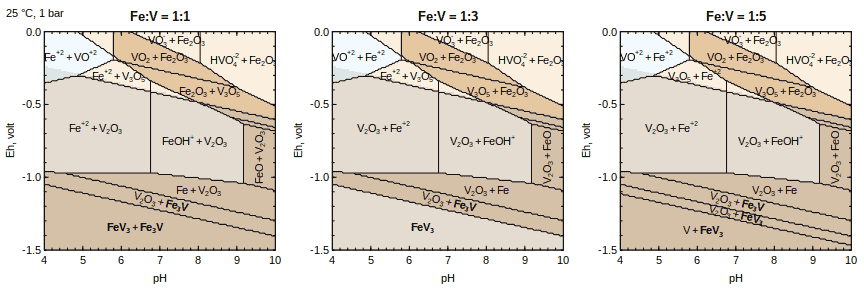In these diagrams, changing the Fe:V ratio affects the fully reduced metallic species. In the 1:1 mixture, the FeV3 + Fe3V assemblage is predicted to be stable instead of FeV. This result is unlike Figure 1 of Singh et al. (2017) but is consistent with the MP page for FeV where it is shown to decompose to this assemblage. On the other hand, FeV3 is stable in the 1:3 mixture. For an even higher proportion of V, the V + FeV3 assemblage is stable, which can be seen for instance in the Pourbaix diagram linked from the MP page for FeV5O12.

Let’s make another diagram for the 1:1 Fe:V composition over a broader range of Eh and pH. The diagram shows a stable assemblage of Fe2O3 with an oxidized bimetallic material, Fe2V4O13.

layout(t(matrix(1:3)), widths = c(1, 1, 0.2))
par(cex = 1)
# Fe-bearing species
basis(c("VO+2", "Fe+2", "H2O", "e-", "H+"))
species(c(iFe.aq, iFe.cr))\$name
species(1:length(iFe.aq), loga.Fe)
aFe <- affinity(pH = c(0, 14, res2), Eh = c(-1.5, 2, res2))
dFe <- diagram(aFe, plot.it = FALSE)
# V-bearing species
species(c(iV.aq, iV.cr))\$name
species(1:length(iV.aq), loga.V)
aV <- affinity(aFe)  # argument recall
dV <- diagram(aV, plot.it = FALSE)
# Bimetallic species
species(iFeV.cr)
aFeV <- affinity(aFe)  # argument recall
dFeV <- diagram(aFeV, plot.it = FALSE, bold = TRUE)
# 1:1 mixture (Fe:V)
a11 <- mix(dFe, dV, dFeV, c(1, 1))
iV2O3 <- info("V2O3")
iFe3V <- info("Fe3V")
iVO4m3 <- info("VO4-3")
iFe2O3 <- info("Fe2O3")
srt <- rep(0, nrow(a11\$species))
srt[a11\$species\$ispecies == paste(iV2O3, iFe3V, sep = ",")] <- -13
srt[a11\$species\$ispecies == paste(iFe2O3, iVO4m3, sep = ",")] <- 90
d11 <- diagram(a11, min.area = 0.01, srt = srt)
water.lines(d11, col = "orangered")

We then compute the affinity for formation of a metastable material, in this case triclinic FeVO4, from the same basis species used to make the previous diagrams. Given the diagram for the stable Fe-, V- and bimetallic materials mixed with the same stoichiometry as FeVO4 (1:1 Fe:V), the difference between their affinities of formation and that of FeVO4 corresponds to the Pourbaix energy difference (-ΔGpbx). This is plotted as a color map in the second diagram. (See the source of this vignette for the code used to make the scale bar.)

# Calculate affinity of FeVO4
species("FeVO4")
aFeVO4 <- affinity(aFe)  # argument recall
# Calculate difference from stable species
aFeVO4_vs_stable <- aFeVO4\$values[] - d11\$predominant.values
# Overlay lines from diagram on color map
diagram(a11, fill = NA, names = FALSE, limit.water = FALSE)
opar <- par(usr = c(0, 1, 0, 1))
col <- rev(hcl.colors(128, palette = "YlGnBu", alpha = 0.8))
image(aFeVO4_vs_stable, col = col, add = TRUE)
par(opar)
diagram(a11, fill = NA, add = TRUE, names = FALSE)
water.lines(d11, col = "orangered")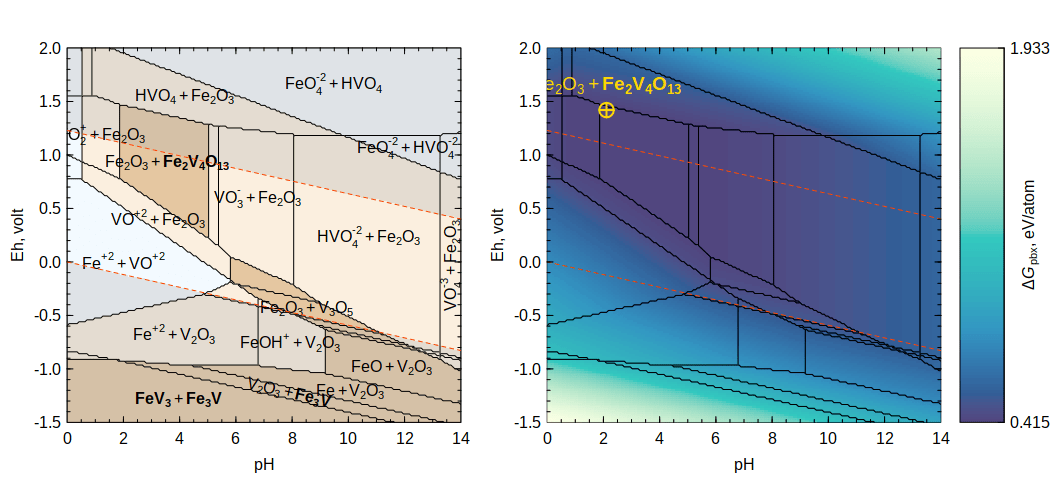Now we locate the pH and Eh that maximize the affinity (that is, minimize ΔGpbx) of FeVO4 compared to the stable species. In agreement with Singh et al. (2017), this is in the stability field of Fe2O3 + Fe2V4O13.

imax <- arrayInd(which.max(aFeVO4_vs_stable), dim(aFeVO4_vs_stable))
pH <- d11\$vals\$pH[imax]
Eh <- d11\$vals\$Eh[imax]
points(pH, Eh, pch = 10, cex = 2, lwd = 2, col = "gold")
stable <- d11\$names[d11\$predominant[imax]]
text(pH, Eh, stable, adj = c(0.3, 2), cex = 1.2, col = "gold")
range(aFeVO4_vs_stable[d11\$predominant == d11\$predominant[imax]])
##  -42.0543 -42.0543

Although one point is drawn on the diagram, FeVO4 has the same Pourbaix energy difference with respect to the entire Fe2O3 + Fe2V4O13 field, as shown by the range() command (the values are dimensionless values of affinity, A/(RT) = -ΔGpbx/(RT)). This can occur only if the decomposition reaction has no free O2 or H2, and means that in this case ΔGpbx in the Fe2O3 + Fe2V4O13 field is equal to the energy above the hull.

To calculate the energy above the hull “by hand”, let’s set up the basis species to be the stable decomposition products we just found. O2 is also needed to make a square stoichiometric matrix (i.e. same number of elements and basis species), but it does not appear in the reaction to form FeVO4 from the basis species. subcrt() is used to automatically balance the formation reaction for 1 mole of FeVO4 and calculate the standard Gibbs energy of the reaction. The convert() command divides this value by -RT (which yields log K of the reaction), showing the same result as calculated above from the Pourbaix diagram. The value of ΔG° in cal/mol (the default for subcrt()) is converted to J/mol, then to eV/mol, and finally eV/atom.

b <- basis(c("Fe2O3", "Fe2V4O13", "O2"))
cal_mol <- subcrt("FeVO4", 1, T = 25)\$out\$G
convert(cal_mol, "logK")
##  -42.0543
J_mol <- convert(cal_mol, "J")
eV_mol <- J_mol / 1.602176634e-19
eV_atom <- eV_mol / 6.02214076e23 / 6
round(eV_atom, 3)
##  0.415

This is equal to the value for the energy above the hull / atom for triclinic FeVO4 on the MP website (0.415 eV, accessed on 2020-11-09 and 2021-02-19). This shows that we successfully made a round trip starting with the input formation energies (eV/atom) from the Materials API, to standard Gibbs energy (J/mol) in the OBIGT database, and back out to energy above the hull (eV/atom).

The concept of using the stable minerals and aqueous species to calculate reaction energetics is formalized in the mosaic() function, which is described next. Because this example modified the thermodynamic data for some minerals that are used below, we should restore the default OBIGT database before proceeding to the next section.

reset()

Mosaic Stacking 1

A mosaic diagram shows the effects of changing basis species on the stabilities of minerals. The Fe-S-O-H system is a common example: the speciation of aqueous sulfur species affects the stabilities of iron oxides and sulfides. Examples of mosaic diagrams with Fe or other single metals are given elsewhere.

A mosaic stack is when predominance fields for minerals calculated in one mosaic diagram are used as input to a second mosaic diagram, where the minerals are now themselves basis species. The example here shows the construction of a Cu-Fe-S-O-H diagram.

First we define the conditions and basis species. It is important to put Cu+ first so that it will be used as the balance for the reactions with Cu-bearing minerals (which also have Fe). Pyrite is chosen as the starting Fe-bearing basis species, which will be changed as indicated in Fe.cr.

logaH2S <- -2
T <- 200
pH <- c(0, 14, res2)
O2 <- c(-48, -33, res2)
basis(c("Cu+", "pyrite", "H2S", "oxygen", "H2O", "H+"))
basis("H2S", logaH2S)
S.aq <- c("H2S", "HS-", "HSO4-", "SO4-2")
Fe.cr <- c("pyrite", "pyrrhotite", "magnetite", "hematite")
Fe.abbrv <- c("Py", "Po", "Mag", "Hem")

Now we calculate affinities for minerals in the Fe-S-O-H system that take account of the changing aqueous sulfur species in S.aq. The result is used to make different layers of the diagram (1 and 2 are both made by the first call to diagram()):

1. Water stability region (gray shading)
2. Predominance fields for the aqueous S species (blue text and dashed lines)
3. Stability areas for the Fe-bearing minerals (black text and lines)
species(Fe.cr)
mFe <- mosaic(S.aq, pH = pH, O2 = O2, T = T)
diagram(mFe\$A.bases, lty = 2, col = 4, col.names = 4, italic = TRUE, dx = c(0, 1, 0, 0), dy = c(-1.5, 0, 1, 0))
dFe <- diagram(mFe\$A.species, add = TRUE, lwd = 2, names = Fe.abbrv, dx = c(0, 0.5, 0, 0), dy = c(-1, 0, 0.5, 0))

Next we load the Cu-bearing minerals and calculate their affinities while changing both the aqueous sulfur species and the Fe-bearing minerals whose stability fields were just calculated. The latter step is the key to the mosaic stack and is activated by supplying the calculated stabilities of the Fe-bearing minerals in the stable argument. This is a list whose elements correspond to each group of changing basis species given in the first argument. The NULL means that the abundances of S-bearing aqueous species are calculated according to the default in mosaic(), which uses equilibrate() to compute the continuous transition between them (“blending”). Because the Fe-bearing minerals are the second group of changing basis species (Fe.cr), their stabilities are given in the second position of the stable list. The result is used to plot the last layer of the diagram:

1. Stability areas for Cu-bearing minerals (red text and lines; orange for chalcopyrite)

After that we add the legend and title.

FeCu.cr <- c("chalcopyrite", "bornite")
Cu.cr <- c("copper", "cuprite", "tenorite", "chalcocite", "covellite")
FeCu.abbrv <- c("Ccp", "Bn", "Cu", "Cpr", "Tnr", "Cct", "Cv")
species(c(FeCu.cr, Cu.cr))
mFeCu <- mosaic(list(S.aq, Fe.cr), pH = pH, O2 = O2,
T = T, stable = list(NULL, dFe\$predominant))
diagram(mFeCu\$A.species, add = TRUE, col = 2, col.names = 2, bold = TRUE, names = FeCu.abbrv, dy = c(0, 0, 0, 0, 0, 1, 0))
col <- c("#FF8C00", rep(NA, 6))
diagram(mFeCu\$A.species, add = TRUE, col = col, lwd = 2, col.names = col, bold = TRUE, names = FeCu.abbrv)
TPS <- c(describe.property(c("T", "P"), c(T, "Psat")), expression(sum(S) == 0.01*m))
legend("topright", TPS, bty = "n")
title("Cu-Fe-S-O-H (minerals only)", font.main = 1)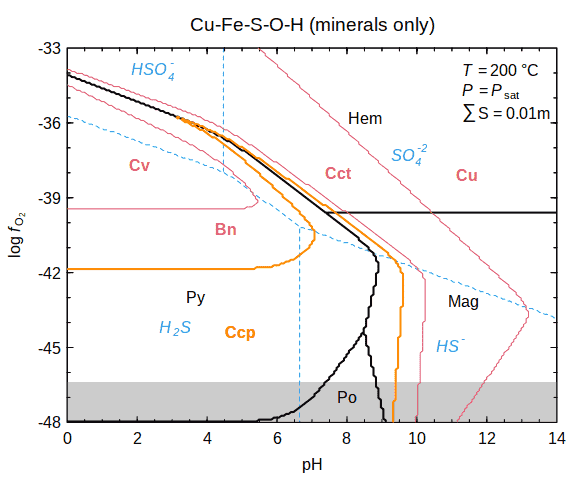This diagram has a distinctive chalcopyrite “hook” surrounded by a thin bornite field. Only the chalcopyrite-bornite reaction in the pyrite field is shown in some published diagrams (e.g. Anderson, 1975; Giordano, 2002), but diagrams with a similar chalcopyrite wedge or hook geometry can be seen in Barton et al. (1977) and Brimhall (1980).

Mosaic Stacking 2

The previous diagram shows the relative stabilities of minerals only. The next diagram adds aqueous species to the system. The position of the boundaries between the stability fields for minerals and aqueous species are calculated for a given activity for the latter, in this case 10-6.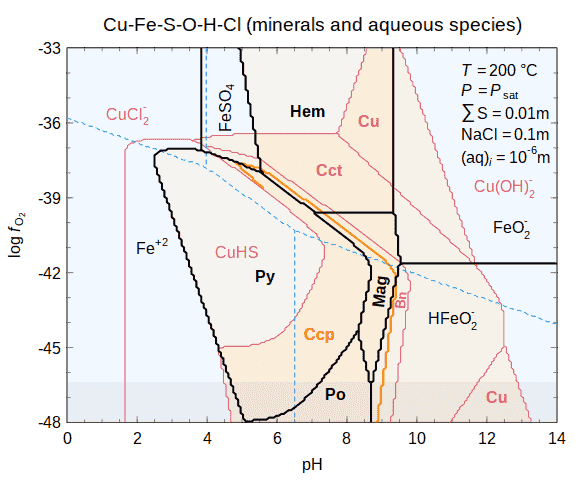After running the code to make this diagram, we can list the reference keys for the minerals and aqueous species.

minerals <- list(Fe.cr = Fe.cr, Cu.cr = Cu.cr, FeCu.cr = FeCu.cr)
aqueous <- list(S.aq = S.aq, Fe.aq = Fe.aq, Cu.aq = Cu.aq)
allspecies <- c(minerals, aqueous)
iall <- lapply(allspecies, info)
allkeys <- lapply(iall, function(x) thermo.refs(x)\$key)
allkeys
## \$Fe.cr
##  "HDNB78" "Ber88"
##
## \$Cu.cr
##  "HDNB78"
##
## \$FeCu.cr
##  "HDNB78" "PK70"
##
## \$S.aq
##  "SHS89" "SH88"
##
## \$Fe.aq
##  "SSH97"  "SSWS97" "SPD+19"
##
## \$Cu.aq
##  "SH88"   "SSH97"  "SSWS97" "AZ01"   "AZ10"

The next code chunk prepends @ to the reference keys and uses the chunk option results = 'asis' to insert the citations into the R Markdown document in chronological order.

allyears <- lapply(iall, function(x) thermo.refs(x)\$year)
o <- order(unlist(allyears))
cat(paste(paste0("@", unique(unlist(allkeys)[o])), collapse = "; "))

Pankratz and King (1970); Helgeson et al. (1978); Berman (1988); Shock and Helgeson (1988); Shock et al. (1989); Sverjensky et al. (1997); Shock et al. (1997); Akinfiev and Zotov (2001); Akinfiev and Zotov (2010); St Clair et al. (2019)

Mixing 2

The previous diagram shows a stability boundary between chalcopyrite and bornite but does not identify the stable assemblages that contain these minerals. This is where mix() can help. Following the workflow described in Mixing 1, we first calculate individual diagrams for Fe-S-O-H and Cu-S-O-H, which are overlaid on the first plot and saved in dFe and dCu. We then calculate the affinities for the bimetallic Cu and Fe minerals and run them through diagram() without actually making a plot, but save the result in dFeCu. Then, we combine the results using mix() to define different proportions of Fe and Cu.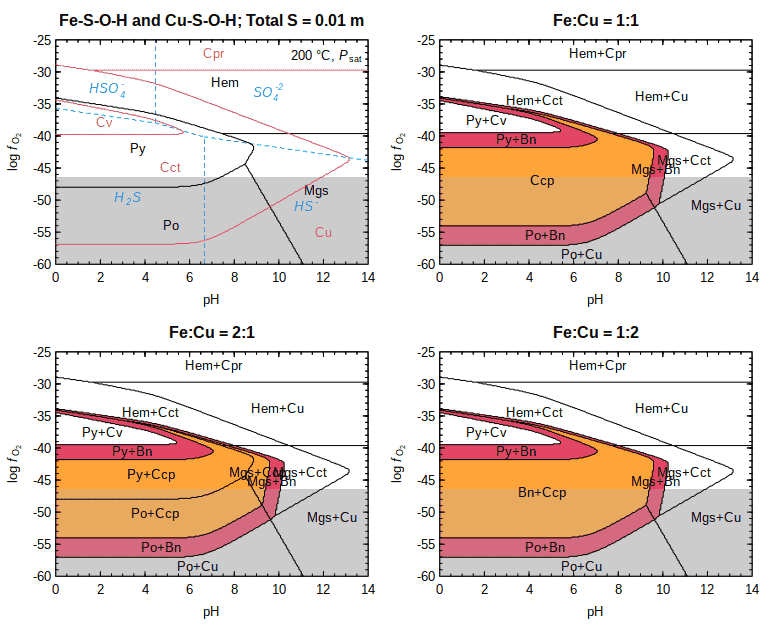These diagrams show that changing the amounts of the metals affects the stability of minerals involved in reactions with chalcopyrite. At a 1:1 ratio of Fe:Cu, chalcopyrite is a stable single-mineral assemblage. At a 2:1 ratio, pyrite, pyrrhotite, or magnetite can coexist in a two-phase assemblage with chalcopyrite. At a 1:2 ratio, an assemblage consisting of the two bimetallic minerals (chalcopyrite and bornite) is stable.

Mosaic Stacking 3

The results of a mosaic stack can also be processed with mash() to label each region with the minerals from both systems. For this example, the speciation of aqueous sulfur is not considered; instead, the fugacity of S2 is a plotting variable. The stable Fe-bearing minerals (Fe-S-O-H) are used as the changing basis species to make the diagram for Cu-bearing minerals (Cu-Fe-S-O-H). Then, the two diagrams are mashed to show all minerals in a single diagram. Greener colors are used to indicate minerals with less S2 and more O2 in their formation reactions.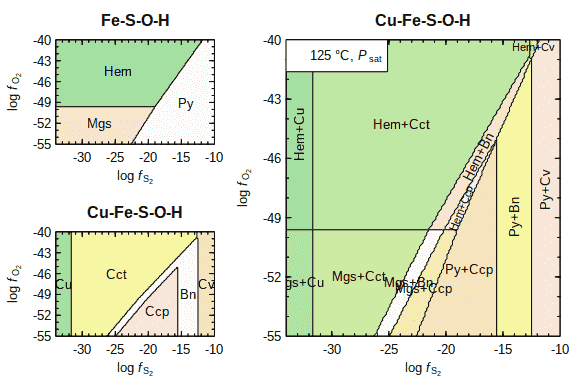The resulting diagram is similar to Figure 2 of Sverjensky (1987); that diagram also shows calculations of the solubility of Cu and concentration of SO4-2 in model Cu ore-forming fluids. The solubility() function can be used to calculate the total concentration of Cu in different complexes in solution (listed in the iaq argument). The bases argument triggers a mosaic() calculation, so that the solubility corresponds that that of stable minerals at each point on the diagram. The pH for these calculations is set to 6, and the molality of free Cl-, which affects the formation of the Cu chloride complexes, is estimated based on the composition of fluids from Table 2 of Sverjensky (1987) (ca. 80000 mg Cl / kg H2O) and the NaCl() function in CHNOSZ. This also gives an estimated ionic strength, which is used in the following mosaic() and affinity() calls to calculate activity coefficients.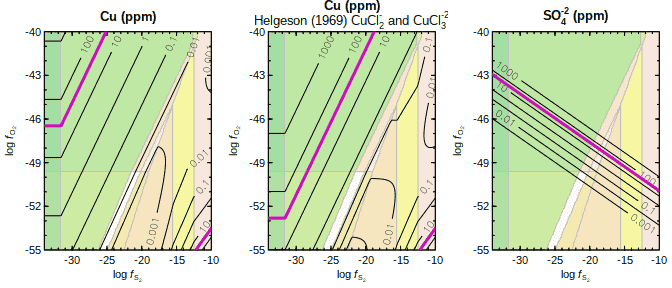After running the code above, we can inspect the value of calc to show the estimated ionic strength and activity of Cl-; the latter is very close to unity.

# Ionic strength
calc\$IS
##  1.86169
# Logarithm of activity of Cl-
log10(calc\$m_Cl * calc\$gam_Cl)
##  0.00584918

The thick magenta lines indicate the 35 ppm contour for Cu and SO4-2. The first plot shows a lower Cu solubility in this region compared to Figure 2 of Sverjensky (1987). The difference is likely due to lower stabilities of Cu(I) chloride complexes in the default OBIGT database, compared to those available at the time (Helgeson, 1969). For the second plot, the standard Gibbs energies of CuCl2- and CuCl3-2 are adjusted so that the logK for their dissociation reactions at 125 °C matches values interpolated from Table 5 of Helgeson (1969). Here are the logK values after the adjustment, followed a reset() call to compare the values with the default database, which is also used for later examples in this vignette. (T was set to 125 above.)

# logK values interpolated from Table 5 of Helgeson (1969)
subcrt(c("CuCl2-", "Cu+", "Cl-"), c(-1, 1, 2), T = T)\$out\$logK
##  -5.2
subcrt(c("CuCl3-2", "Cu+", "Cl-"), c(-1, 1, 3), T = T)\$out\$logK
##  -5.6
# Default OBIGT database
reset()
subcrt(c("CuCl2-", "Cu+", "Cl-"), c(-1, 1, 2), T = T)\$out\$logK
##  -4.75568
subcrt(c("CuCl3-2", "Cu+", "Cl-"), c(-1, 1, 3), T = T)\$out\$logK
##  -3.35596

The higher stability of these complexes from Helgeson (1969) causes the 35 ppm contour to move closer to the position shown by Sverjensky (1987).

Interestingly, the calculation here also predict substantial increases of Cu concentration of Cu at high fS2 and low fO2, due to the formation of bisulfide complexes with Cu. The aqueous species considered in the calculation can be seen like this:

names(iCu.aq)
##   "Cu+2"     "CuCl3-2"  "CuCl+"    "CuCl2"    "CuCl3-"   "CuCl4-2"
##   "CuOH+"    "CuO"      "HCuO2-"   "CuO2-2"   "Cu+"      "CuOH"
##  "Cu(OH)2-" "CuHS"     "Cu(HS)2-" "CuCl"     "CuCl2-"

CuHS and Cu(HS)2- can be excluded by removing S from the retrieve() call above (i.e. only c("O", "H", "Cl") as the elements in possible ligands); doing so precludes a high concentration of aqueous Cu in the highly reduced, sulfidic region.

The third plot for the concentation of SO4-2 is simply made by using affinity() to calculate the affinity of its formation reaction as a function of fS2 and fO2 at pH 6 and 125 °C, then using solubility() to calculate the solubility of S2(gas), expressed in terms of moles of SO4-2 in order to calculate parts per million (ppm) by weight.

Secondary Balancing

Predominance diagrams in CHNOSZ are made using the maximum affinity method, where the affinities of formation reactions of species are divided by the balancing coefficients (Dick, 2019). Usually, these balancing coefficients are taken from the formation reactions themselves; for example, if they are the coefficients on the Fe-bearing basis species, then the reactions are said to be “balanced on Fe”.

Some diagrams in the literature are made with secondary balancing constraints in addition to the primary ones. For example, reactions of Fe-bearing minerals are balanced on Fe, and reactions of Cu-bearing minerals are balanced on Cu; these are both primary balancing coefficients. Then, reactions between all minerals are balanced on H+ as the secondary balancing coefficients. The core concept is to apply the secondary balance while also maintaining the primary balance; a method to do this has been implemented in the rebalance() function.

Different parts of the script to make the diagrams are described below; press the button to show the entire script.

We first define basis species to contain both Cu- and Fe-bearing species. The axis is the ratio of activities of Fe+2 and Cu+; the label is made with ratlab().

basis(c("Fe+2", "Cu+", "hydrogen sulfide", "oxygen", "H2O", "H+"))
xlab <- ratlab("Fe+2", "Cu+")

We then calculate the diagrams for the primary balancing coefficients, for the groups of only Fe-, only Cu-, and only Fe+Cu-bearing minerals. It is obvious that the first two systems are balanced on Fe and Cu, respectively, but the third has a somewhat unusual balance: H+. See Reaction 4 of McKenzie and Helgeson (1985) for an example.

# Only Fe-bearing minerals
species(c("pyrite", "pyrrhotite", "magnetite", "hematite"))
aFe <- affinity("Fe+2" = c(0, 12), O2 = c(-40, -16), T = 400, P = 2000)
names <- info(aFe\$species\$ispecies)\$abbrv
dFe <- diagram(aFe, xlab = xlab, names = names)
title(bquote("Only Fe; 1° balance:" ~ .(expr.species(dFe\$balance))))
label.plot("A")

# Only Cu-bearing minerals
species(c("covellite", "chalcocite", "tenorite", "cuprite"))
aCu <- affinity(aFe)  # argument recall
names <- info(aCu\$species\$ispecies)\$abbrv
dCu <- diagram(aCu, xlab = xlab, names = names)
title(bquote("Only Cu; 1° balance:" ~ .(expr.species(dCu\$balance))))
label.plot("B")

# Only Fe- AND Cu-bearing minerals
species(c("chalcopyrite", "bornite"))
aFeCu <- affinity(aFe)
names <- info(aFeCu\$species\$ispecies)\$abbrv
dFeCu <- diagram(aFeCu, xlab = xlab, balance = "H+", names = names)
title(bquote("Only Fe+Cu; 1° balance:" ~ .(expr.species(dFeCu\$balance))))
label.plot("C")

Now comes the secondary balancing, where all reactions, not only that between bornite and chalcopyrite, are balanced on H+. We first rebalance the diagrams for the Fe- or Cu-bearing minerals to make diagram D. Note that after secondary balancing with rebalance(), the argument balance = 1 should be used in diagram() to prevent further balancing. This is because rebalance() preserves the primary balancing for Fe- and Cu-bearing minerals (internally the “plotvals” components of dFe and dCu).

Then we rebalance diagrams D and C to make the final diagram in E. The fields in this diagram are labeled with mineral abbreviations from the OBIGT database.

# Fe- or Cu-bearing minerals
ad1 <- rebalance(dFe, dCu, balance = "H+")
d1 <- diagram(ad1, xlab = xlab, balance = 1, names = names)
title(bquote("Only Fe or Cu; 2° balance:" ~ .(expr.species("H+"))))
label.plot("D")

# All minerals
d1\$values <- c(dFe\$values, dCu\$values)
ad2 <- rebalance(d1, dFeCu, balance = "H+")
diagram(ad2, xlab = xlab, balance = 1, names = names)
title(bquote("Fe and/or Cu; 2° balance:" ~ .(expr.species("H+"))))
label.plot("E")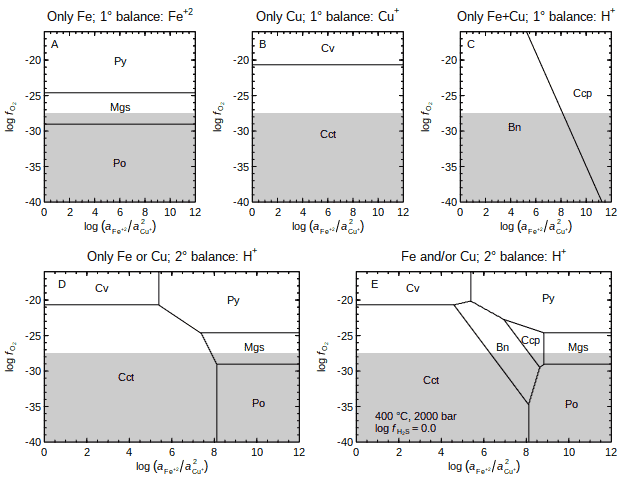The final diagram is like one shown in Figure 5 of Brimhall (1980) and Figure 5 of McKenzie and Helgeson (1985).

Challenge: Although the diagram here is drawn only for H2S in the basis species, take it a step further and make a mosaic diagram to account for the stability of HSO4- at high oxygen fugacity.

Other Possibilities

Conceptually, the methods described above treat different metal-bearing elements as parts of distinct chemical systems that are then joined together. Other methods may be more suitable for considering multiple metals (or other elements) in one system.

Balancing on a Non-Metal

As shown in the secondary balancing example, there is no requirement that the balancing coefficients come from a metal-bearing species. It is possible to make diagrams for minerals with different metallic elements simply by using a non-metallic element as the primary balance. Here is an example for the Cu-Fe-S-O-H system. The reactions are balanced on O2, which means that no O2 appears in the reaction between any two minerals, but Fe+2 and/or Cu+ can be present, depending on the chemical composition. Saturation limits are shown for species that have no O2 in their formation reactions.

basis(c("Fe+2", "Cu+", "hydrogen sulfide", "oxygen", "H2O", "H+"))
basis("H2S", 2)
species(c("pyrite", "magnetite", "hematite", "covellite", "tenorite",
"chalcopyrite", "bornite"))
a <- affinity("Cu+" = c(-8, 2, 500), "Fe+2" = c(-4, 12, 500), T = 400, P = 2000)
names <- info(a\$species\$ispecies)\$abbrv
d <- diagram(a, xlab = ratlab("Cu+"), ylab = ratlab("Fe+2"), balance = "O2", names = names)
title(bquote("Cu-Fe-S-O-H; 1° balance:" ~ .(expr.species(d\$balance))))
species(c("pyrrhotite", "ferrous-oxide", "chalcocite", "cuprite"))
asat <- affinity(a)  # argument recall
names <- asat\$species\$name
names <- "ferrous oxide"
diagram(asat, type = "saturation", add = TRUE, lty = 2, col = 4, names = names)
legend("topleft", legend = lTP(400, 2000), bty = "n")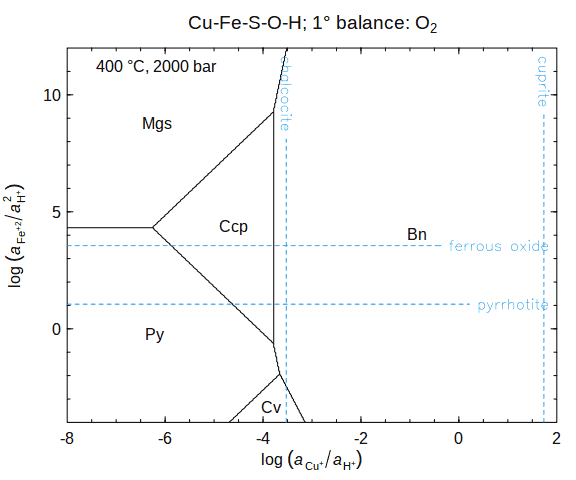This example was prompted by Figure 3 of McKenzie and Helgeson (1985); earlier versions of the diagram are in Helgeson et al. (1969) and Helgeson (1970).

In some ways this is like the inverse of the mosaic stacking example. There, reactions were balanced on Fe or Cu, and fO2 and pH were used as plotting variables. Here, the reactions are balanced on O2 and implicitly on H+ through the activity ratios with aFe+2 and aCu+, which are the plotting variables.

More common diagrams of this type are balanced on Si or Al. See demo(saturation) for an example in the H2O-CO2-CaO-MgO-SiO2 system.

Mosaic Combo

Instead of adding minerals with different metals by stacking mosaic diagrams, it may be possible to include two different metals in the basis species and formed species. The mosaic() and equilibrate() functions can be combined to balance on two different elements. The example here compares two methods applied to N-, C-, and N+C-bearing species because bimetallic aqueous species are not currently available in the OBIGT database. The total activities used here are modified from the example for sedimentary basin brines described by Shock (1993), which is also the source of the thermodynamic parameters for acetamide.

1. The “effective equilibrium constant” (Keff) method (Robinson et al., 2021) is used to calculate the activity of acetamide for a total activities of neutral and ionized species, i.e. ∑(ammonia and ammonium) and ∑(acetic acid and acetate).

2. Using the mosaic combo method, the mosaic() command calculates equilibrium activities of NH3 and NH4+ for a given total activity of N in the basis species, and calculates the corresponding affinities of the formed species. Then, the equilibrate() command calculates equilibrium activities of the formed species for given total activity of C, and combines them with the activities of the changing basis species (NH3 and NH4+).

The mosaic combo method (solid black line) produces results equivalent to those of the Keff method (dashed blue line).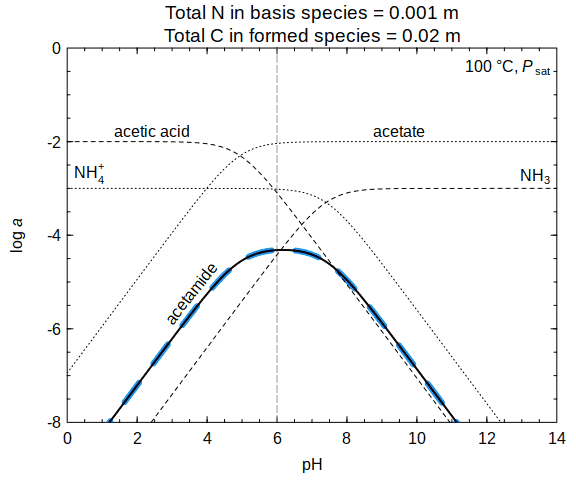The diagram shows the ionization of acetic acid and NH3 at different pHs. The predicted appearance of acetamide (CH3CONH2) is a consequence of the interaction between the N-bearing and C-bearing species, and is analogous to the formation of a bimetallic complex.

Thanks to Kirt Robinson for the feature request and test case used in this example.

Document History

• 2020-07-15 First version.
• 2021-03-01 Improve mineral abbreviations and placement of labels; use updated DFT energies from Materials Project; add Mosaic Stacking 2 (minerals and aqueous species); add Keff calculation; add ΔGpbx color scale.